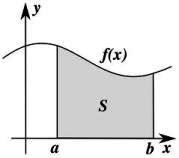## August 28, 2010

### A mathematical look at change can be helpful for practical change professionals

A few months ago, mathematics professor Steven Strogatz wrote two columns in the New York Times called Change We Can Believe In and It Slices, It Dices. The columns are about calculus, a branch in mathematics focused on limits, functions, derivatives, integrals, and infinite seriesCalculus is the mathematics of change. Its two basic branches are differential calculus and integral calculus. Without giving an in-depth explanation of these two topics, here is a brief introduction.

Differential calculus
is the study of the derivative of functions. Calculating the derivative is called differentiation. The derivative tells you how fast something is changing, how far you’re going up or down a slope for every step you take. The derivate is the approximation to the slope of a graph. The derivate can be calculated for each point of a function but also for every point which leads to the derivate function (see picture from www.derivate.it). When a slope is going up its derivate is positive, when a slope is going down it's negative. At the peak and the bottom of a curve it is zero. At those points, change momentarily stands still.Integral calculus
is the study of integrals which tells you how much something is accumulating. It is the calculus of summation. Calculating the integral is measuring the area under a curve (see picture on the right). Functions can have some very irregular shapes which can make it quite hard to easily measure this area under the curve. What integral calculus does to solve this problem, is slice that area up into thin slices, calculate the volume of the slices and then cleverly adding them up again. On a side note, integrating is the inverse operation of differentiation.

This is all very well, but is this of any relevance for practical change professionals? I believe it is. As I wrote before, my observation is that people involved in change in organizations, like consultants, project managers, line managers, coaches, sometimes get discouraged about how change is proceeding. Slightly changing your perspective on the change results can be very helpful in such instances. In my post Visualizing progress: expect fluctuation and watch the trend line I explained this as follows.Progress hardly ever happens in a straight line. The picture on the right shows a real life example of an improvement process. The red line shows the actual values found (for instance the sales at a certain point in time). As you see, the levels constantly fluctuate. The blue line is the trend line which shows that over time there is a slow but steady improvement. The arrows show the following: Arrow 1: fast first results, quick progress. Arrow 2: rather heavy fall back. Arrow 3: quick improvement again. Arrow 4: serious fall back again after which improvement picks up again. It would be very easy to get discouraged when focusing too much on the fluctuations, at point 2 and 4 for instance. Two things are important to remember: 1) It is normal for progress to show this kind of fluctuation, and 2) The trend line is an important line to watch. This line shows you that there is actual growth overall. The trend line is a very motivating line to watch.

Strogatz' points are valuable additions to my previous post. Applying the explanations of derivates and integrals to my original graph you get the picture on the right. Let's apply them to the potentially most depressing point of the graph which is point 4. The red line shows the derivate of point 4, the colored areas show the integral of the graph until point 4. Both give reason for a positive and hopeful view on the change results. The derivate (red line) at point 4 the negative slope is getting less steep which says things are still getting worse but this decline happens more slowly which gives hope that the bottom is in sight and a turn to a positive slope may be near. The colored areas show the integral which, the area under the curve. The red area visualizes the accumulation of everything that has been achieved from point 0 to point 3. As you see it is all below 0 so the result is negative. This blue area visualizes the accumulation of everything that has been achieved from point 3 to point 4 and the absolute value is reaching 0 again, the Nett result of the change process is positive because the blue area is much larger than the red area. A lot has been achieved. The thought, at point 4, that every effort has been in vain is therefore unjustified.